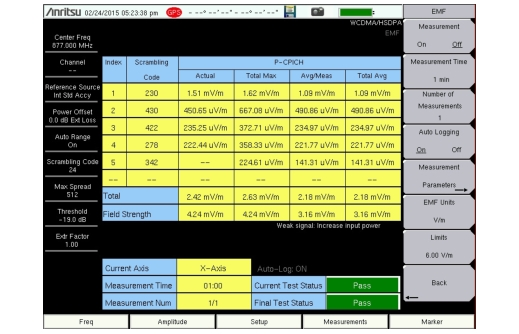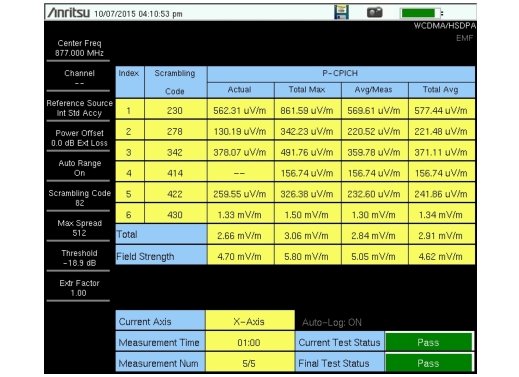Electromagnetic Field (EMF) MeasurementsOption 444 : W-CDMA OTA : Measurement Results (W-CDMA)

Measurement Results (W-CDMA)
The measurement starts by setting the antenna’s X axis and capturing over-the-air data. If a sync signal is found and there is a valid scrambling code, the PCPICH and Channel Power in 5 MHz bandwidth are stored. This is repeated for Y and Z axes. If any one of the axes has a valid scrambling code, the isotropic result (for example, (PCPICH2X + PCPICH2Y + PCPICH2Z)0.5) for each of the above parameters is displayed as the Actual result.
The PCPICH Actual, Total Max, and Avg/Meas parameters are displayed as fixed columns in the measurement results table. See Figure: W-CDMA EMF Measurement Results. Total Max is the max value computed from all measurements completed thus far within the measurement period (Measurement Time × Number of Measurements). Avg/Meas is the running average of the isotropic results computed from all measurements completed thus far within the specified Measurement Time.
Using the Display Params submenu, you can select the computed result to display in the rightmost table column. Refer to Display Menu.There is no axis dwell time parameter. If a sync signal is not found within a specific time, data for the current axis is excluded and the instrument switches to the next axis.
The Field Strength number is the measured Channel Power in a 5 MHz bandwidth.
If the extrapolation factor is turned On, the Emax value is the extrapolated PCPICH for Total Max, Total Min, Total Avg, or Avg/Meas, and is computed as follows:
Emax = Epcpich × √k
where Epcpich is the root sum square (rss) value of the common pilot signal recorded in each axis and k is the extrapolation factor provided by the network operator. For example:
Emax (pcpich total max) = Epcpich total max × √k

 Note The extrapolation factor k is the ratio of the maximum total output power at the base station to the power of PCPICH at the base station. If there is a power boosting factor (BF), k = (max total output power ÷ P(PCPICH)) ÷ BF.Changing the extrapolation factor immediately updates the displayed values, except for Field Strength. Refer to Meas Params Menu (W‑CDMA).
If a valid scrambling code is obtained even once during the entire measurement period, an entry is made in the table. A maximum of 6 scrambling codes can be detected. The Total row sums the isotropic numbers for the selected display parameter across scrambling codes.
If no valid scrambling code is detected for any of the three axes, the isotropic numbers are excluded from all measurement results (Total Max, Total Min, Total Avg, Avg/Meas). In this case, the display shows “--”.
Pass/Fail
The limit check is performed at the end of each Measurement Time. If the Field Strength (Avg/Meas) exceeds the set limit, the Current and Final Test Status are marked as Fail in red. If the Field Strength (Avg/Meas) does not exceed the limit, the Current Test Status is marked as Pass in green. In the example in Figure: W-CDMA EMF Measurement Display, the Field Strength (Avg Meas) is 5.05 mV/m.
If all of the measurements pass, the Final Test Status is updated to Pass in green.